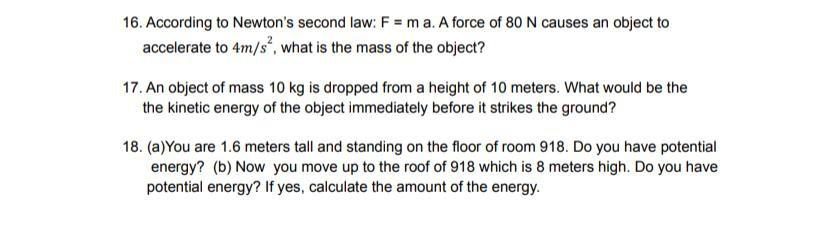Question:

# 16. According to Newton's second law; F = m a. A force of 80 N causes an object to accelerate to 4m/s", what is the mass of the16. According to Newton's second law; F = m a. A force of 80 N causes an object to accelerate to 4m/s", what is the mass of the object? 17. An object of mass 10 kg is dropped from a height of 10 meters. What would be the the kinetic energy of the object immediately before it strikes the ground? 18. (a) You are 1.6 meters tall and standing on the floor of room 918. Do you have potential energy? (b) Now you move up to the roof of 918 which is 8 meters high. Do you have potential energy? If yes, calculate the amount of the energy.